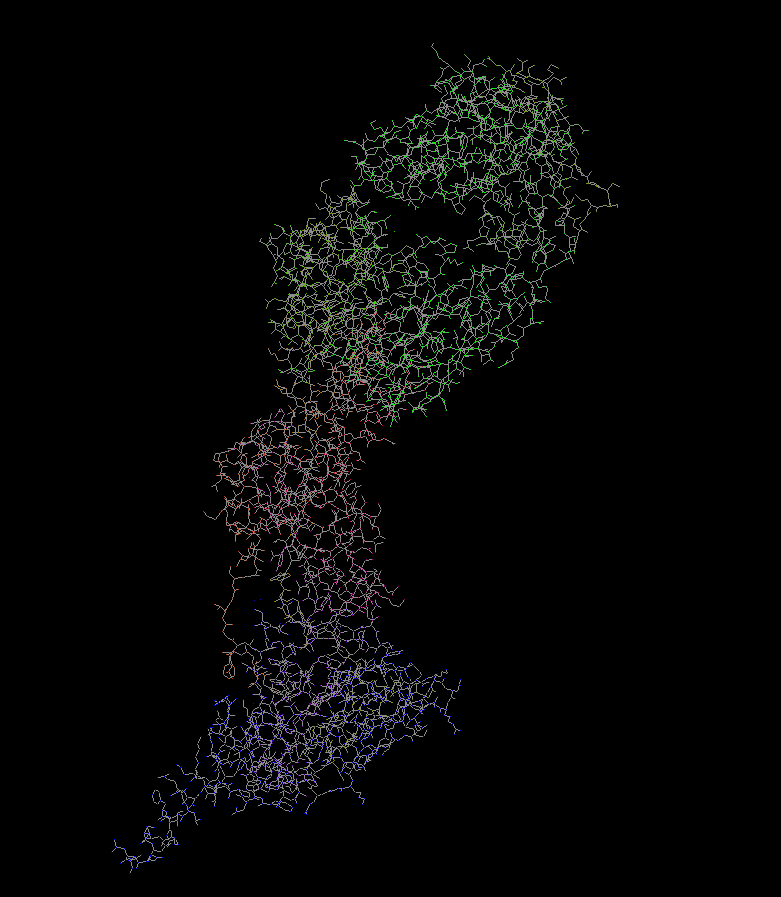labbook

### Bresenham's line algorithm in rust

Yesterday, I implemented Bresenham’s line algorithm for the n-th time.

It’s for my molecule viewer, working title: laurel. Here’s some progress posts.Here, you can see a protein model (pdb id: 4hjw) where the bonds are rendered using this line algorithm. (post)

To keep myself from reinventing the correct and nice way to implement this algorithm, I make this note here.

For quick reference, here is my way of doing it as of the date of publishing.

``````fn line(screen: &mut Screen, x0: u32, y0: u32, x1: u32, y1: u32, px: [u8; 4]) {
let dx = i32::abs(x1 as i32 - x0 as i32);
let dy = i32::abs(y1 as i32 - y0 as i32);
let sx = if x0 < x1 { 1 } else { -1 };
let sy = if y0 < y1 { 1 } else { -1 };

let mut error = if dx > dy { dx } else { -dy } / 2;
let mut x = x0 as i32;
let mut y = y0 as i32;
loop {
screen.set_pixel(x as u32, y as u32, px);

if x == x1 as i32 && y == y1 as i32 {
break;
}

let e = error;
if e > -dx {
error -= dy;
x += sx;
}
if e < dy {
error += dx;
y += sy;
}
}
}
``````